delphi equation equation equation solver equation evaluator equation editor for equation solving solve equation equation source equation editor equation control equation grapher graph equation
Инструменты Разработчика :: Прочее
Игры :: Детские Игры
Дом и Обучение :: Математика## Qds Equations

Редактор формул Qds Equations™ компонент Delphi, позволяющий вводить и отображать формулы.

Ключевые слова:
delphi equation, delphi formula, delphi integral, delphi greek, delphi limit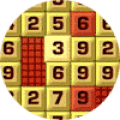## Arithmetic Game

Pick numbers to complete the equation.

Ключевые слова:
arithmetic, mathematics, calculation, equation, mathematics games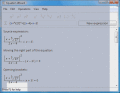## Equation Wizard

The program automatically solves algebraic equations of any order written in any form. Enter your equation and click just one button! Step by step the program will solve the equation, find its roots and describe all its operations.

Ключевые слова:
equation, calculator, math## Chemical Equation Expert

Chemical Equation Expert is an integrated tool for chemistry professionals and students. You'll find complicated work such as balancing chemical equations and related calculations so easy and even enjoyable!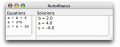## AutoAbacus

AutoAbacus is a powerful equation solving library that finds solutions to equation sets with a snap. A set of equations can be passed in as text, while AutoAbacus attempts to find a solution that satisfies all constraints.

Ключевые слова:
autoabacus, java, business rules, rules engine, math## Easy Equation Solver

Easy Equation Solver is a simple and easy to use program for solving equations. The program can solve: Quadratic Equations, Cubic Equations, 2 Equations with 2 Unknowns, 3 Equations with 3 Unknowns, Determinants 2x2 and Determinants 3x3.

Ключевые слова:
equation solver, quadratic, cubic, unknowns, equations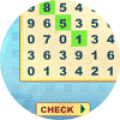## Math Search

Solve the equation and search for the answer.

Ключевые слова:
math search, word search, math games, mathematics games, word games## Math-o-mir

Basically an equation editor, however not focused over one single equation, but you can write your mathematical artwork over several pages. Illustrate your equations using hand-drawing tools. Use symbolic calculator and function plotter.

Ключевые слова:
equation editor, math, notepad, symbolic computation, function plotter## WebCab Functions for Delphi

Add refined numerical procedures to either construct a function of one or two variables from a set of points (i.e. interpolate), or solve an equation of one variable; to your .NET, COM and XML Web service Applications. Delphi 3-8 & 2005 are supported

Ключевые слова:
interpolation, extrapolation, Delphi, .NET, COM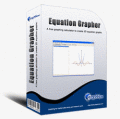## Equation Grapher

Equation grapher is a free graphing calculator to graph equations. Equation Grapher is a free graphing calculator to graph equations. Equation Grapher is a free graphing calculator to graph equations.

Ключевые слова:
equation grapher, free graphing calculator, graph equation, graph equations

Страница: 1 | 2 | 3 | 4 | 5
 Категории: Главная Аудио Бизнес Рабочий Стол Инструменты Разработчика Игры Дом и Обучение Интернет Мультимедия Утилиты Видео Вёб Разработка Свежий софт: Stockalyze TS School Hide.me VPN for Windows Account Hacker Email Hacker